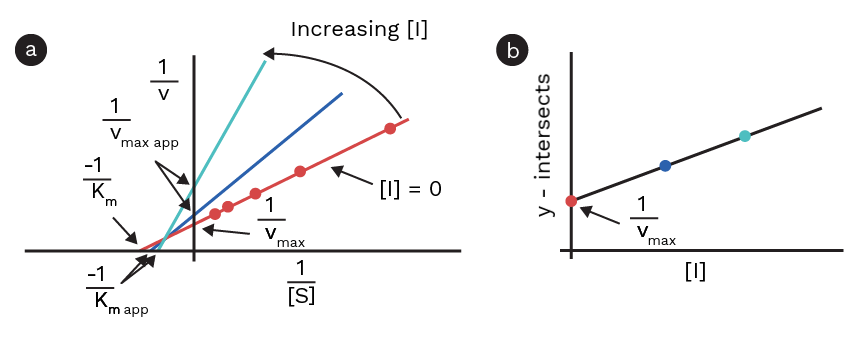A mixed inhibitor interacts with the enzyme alone and with the enzyme-substrate complex. The double-reciprocal equation for mixed inhibition is as follows:

1/V0 = α’/Vmax + km•α/Vmax • 1/[S]

where α = 1+[I]/kI and α’ = 1+[I]/k’I

For mixed inhibition, the Lineweaver-Burk plots show both different slopes and different y-intercepts at different inhibitor concentrations. To calculate the parameters, in this case, 2 new plots must be prepared: first, plot the intercepts against the inhibitor concentrations; this makes it possible to obtain K’I and Vmax, as described under uncompetitive inhibition. Second, plot the slopes against the inhibitor concentrations; from this, KI can be found. The slope of this plot is km / Vmax, therefore multiplying this slope with Vmax already obtained gives Km.Figure 1: a) Lineweaver-Burk plot showing the mixed inhibition. b) Shows the y-intercepts of each linear regression plotted against the inhibitor concentration.

## Noncompetitive inhibition

In the special case of mixed inhibition where α = α', i.e., KI = K'I, the type of inhibition is called noncompetitive inhibition. In this special case, the inhibitor interacts in a favorable manner with the enzyme-substrate complex as it does with the enzyme alone. When plotting kinetic data in a Lineweaver-Burk plot, a common x-intercept shows that the competitor is noncompetitive [1,2].

The double-reciprocal equation for noncompetitive inhibition is thus as follows:

1/V0 = α/Vmax + km•α/Vmax • 1/[S]

where α = 1+[I]/kI

When plotting kinetic data using a noncompetitive inhibitor, the apparent Km remains the same as the actual Km, and it can be calculated from a Lineweaver-Burk plot by dividing the slope with the y-intercept . To calculate Vmax and KI, the y-intercepts of the different lines obtained from linear regression of Lineweaver-Burk plots at different inhibitor, concentrations must be plotted against the inhibitor concentration. When fitted using linear regression, Vmax and KI can be calculated from this plot in the same manner as that in the case of uncompetitive inhibition: Vmax is calculated by taking the reciprocal to the y-intercept of this line, and KI is calculated by dividing the y-intercept with the slope.

## Steps of calculating the kinetic parameters when using a noncompetitive inhibitor

• Prepare Lineweaver-Burk plots of the kinetic data and fit the data using linear regression (1 fit per inhibitor concentration).
• Calculate Km by dividing the slope of any of these lines with the corresponding y-intercept (Km obtained should not depend on the line used).
• Plot the y-intercepts of each of these fits as a function of the inhibitor concentration.
• To calculate Vmax, take the reciprocal of the y-intercept of this plot.
• To calculate KI, divide the y-intercept of this plot with the slope.
1. Lehninger, Albert L.; Nelson, David L.; Cox, Michael M. (2008). Principles of Biochemistry (5th ed.). New York, NY: W.H. Freeman and Company. ISBN 978-0-7167-7108-1.

2. Atkins, Peter W.; de Paula, Julio; Friedman, Ronald (2009). Quanta, Matter, and Change: A molecular approach to physical chemistry. Oxford University Press. ISBN 978-0-19-920606-3.

Theory overview